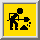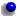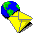# Translated by Flora R. Levin###Return to Murrey Math##Introduction -- Nichomachus of Gerasa

• Nichomachus was a Syrian Greek who was supremely eminent in mathematics, Pythagorean philosophy, arithmology, and harmonics.

• He was motivated by the two main aspects of Pythagorean thought -- mathematics and theology. This Pythagorean ideal led to the development of a mathematically dominated religion. Geometry and arithmetic were considered to be incontrovertible tools of the sister sciences -- astronomy and harmonics (i.e., music).

• The Quadrivium was a curriculum of four subjects -- arithmetic, geometry, spherics (astronomy), and harmonics (music) -- which were linked together by being described solely in terms of number.
• Arithmetic was the study of number absolute.
• Geometry was the study of the number at rest.
• Astronomy was the study of the number in motion.
• Harmonics (music) was the study of applied arithmetic.

• Arithmology involved two kinds of mental activity -- the mathematical and mystical insight.

• Wisdom was a term applied solely to the knowledge of the truth -- truth eternal and exact that inheres in the world of ideas as opposed to the world of illusory appearances. Philosophy was the desire for and the pursuit of wisdom.##Chapter 1

### The book is a manual outlining the doctrine of harmonics

• He is writing this manual while traveling.##Chapter 2

### On the two species of the voice, the intervallar and the continuous, and their regions

• There are two species of the human voice which are subsumed under the genus motion
• Intervallar (melody)
• Stops on every note and renders the change in all the parts perceptible
• Is under nature's control and is limited by different operations

• Continuous (talking)
• Is by nature indeterminate in its compass
• Is regulated by the speaker

• The Pythagoreans considered motion to be the physical, observable, and measurable cause of sound. Space was viewed as an absolute dimension subject solely to the laws of mathematics.

• Melodic space is purely mathematical; its dimensions absolute, fixed, and unchangeable. Within this absolute space there is a place for each note of the scale. Because each of these places is fixed by the laws of mathematics, it must be the true location of any pitch. These absolute places for pitch form the coordinates of the Pythagorean-Platonic universe. In the Timaeus Plato demonstrated that this universe extends over four octaves and a major sixth, and comprises one genus only -- the diatonic.

• The mathematics of the Pythagoreans had one object -- the uniform and permanent universe of the quantitative.##Chapter 3

• ### Among objects of perception, the music of the planets is considered to be the prototype of our music according as we imitate it

• It is probable that the names of the notes were drived from the seven stars which traverse the heavens and travel around the earth. All swiftly whirling bodies necessarily produce sounds. These sounds differ from one another because the planets differ in size, speed, and position.

• The planets are related to notes in the following manner:  Planet Name of Note Pitch Kronos (Saturn; Phainon, "shining one") hypate (highest up) E Zeus (Jupiter; Phaethon, "brilliant one") parhypate F Ares (Mars; Pyroeis, "fiery one") lichanos or hypermese G Helios (Sun) mese (middle) A Hermes (Mercury; Stilbon, "gleaming one") paramese or trite B flat Aphrodite (Venus; Phosphoros, "light bearer") paranete C Selene (Moon) nete (lowest) D

• This scale structure covers the total range of a minor seventh or one whole tone less than an octave.

• Nichomachus' system is a geocentric one in which the distances of the planets are calculated from the Earth as the center of the universe.##Chapter 4

• ### The properties in musical notes are regulated by number

• Among string instruments, the greater and more powerful the tension, the greater and higher pitched are the notes produced; while the lesser tensions produce more slow moving and lower pitched notes.

• In wind instruments, the greater bores and greater lengths produce a slow moving and relaxed note.

• All these factors are governed by number. For quantity is held to be the property of number and number alone.##Chapter 5

• ### Pythagoras, by adding the eighth string to the seven-stringed lyre, instituted the attunement of the octave

• The heptachord, the seven-stringed lyre, was invented by Terpander. Pythagoras added an eighth string in order to extend the reach of the instrument to a full octave. He inserted a whole tone between two tetrachords.

• For Pythagoras, the word harmonia meant "octave" in the sense of an "attunement" which manifests within its limits both the proper "fitting together" of the concordant intervals (fourth and fifth) and the difference between them (the whole tone).

• Whatever can be said of any one octave holds true for all octaves.

• The hemiolic ratio [3:2] of the fifth is found to be a system composed of the epitritic ratio [4:3] and of the sesquioctave ratio [9:8]. The whole-tone is then in a sesquioctave ratio.

(3 : 2) / (4 : 3) = (9 : 8)##Chapter 6

• ### How the numerical proportions of the notes were discovered

• As the story goes, Pythagoras walked by a smithy and heard the musical sounds ringing out from the anvil. The intervals which were pleasing to the ear were the octave, the fifth, and the fourth.

• The weights of the hammers were responsible for the differences in pitch which he heard. The weights which were used were 12, 9, 8, and 6.
• An octave was produced by the weights of 12 and 6 -- [2:1].
• A fifth was produced by the weights of 12 and 8 -- [3:2].
• A fourth was produced by the weights of 12 and 9 -- [4:3].
• Discordant whole tone was produced by the weights of 9 and 8 -- [9:8].

• Pythagoras invented a new eight-string lyre which was called the Dorian octachord.

• The relation of tension to frequency of vibration is expressed by 4:1 (octave), 9:4 (fifth), and 16:9 (fourth).

• At the very center of Pythagorean thought lies the idea of the corporeality of number. They conceived of numbers as having material substance.##Chapter 7

• ### On the division of the octave in the diatonic genus

• Thus Pythagoras discovered the progression in this diatonic genus from the lowest note to the hightest note.

• Pythagoras was able to prove that any two tetrachords whose extemes are in the proportion 4:3 and which are separated from one another by a whole tone in the proportion 9:8, add up to an octave:
4:3 x 4:3 x 9:8 = 144:72 or 2:1

• He could also prove that the fifth on his Dorian octachord, when added to a founth, resulted in an octave:
3:2 x 4:3 = 12:6 or 2:1

• Within the framework of an octave, there is a mathematically ordained place for the fourth, for the fifth, and for the whole tone. All the ratios involved in the structure of the octave are comprehended by a single construct -- 12:9::8:6. This construct came to constitute the essential paradigm -- of unity from multiplicity, of concinnity between opposites, of truth incarnate.##Chapter 8

• ### Explanation of the references to harmonics in the Timaeus

• In providing the mathematical basis for the musical concords, Pythagoras had made a fundamental contribution to the mathematization of music.

• In numerical terms, the leimma -- the "remaining" interval or semi-tone -- has the value of 256:243.

• Plato constructed two geometric progressions in whihc the ratios between the terms are
• 2:1 -- 1, 2, 4, 8
• 3:1 -- 1, 3, 9, 27

• Plato combined these two progressions which produced a seven-termed series:
1, 2, 3, 4, 8, 9, 27

• It was traditional to prepresent these two progressions by a diagram in the shape of a Lambda.

• Plato found the harmonic mean, b = [2ac]/[a+c], and the arithmetic mean, b = [a+c]/2, to provide the "middle" notes in the octave. Plato obtained the following terms which generated two octaves:
 4/3 3/2  8/3   9/2 16/3 6   27/2 18 

• Plato's calculations showed that there was no center to the octave, no halving of the whole tone, no perfect union of opposites, and no rationality to the cosmos. This had to be hidden from view.##Chapter 9

• ### The evidence of Philolaus concerning our statements

• The greatest discovery of Pythagoras was that the pitch produced by a plucked string depends upon the length of the string, and that strings whose lengths are to each other as the ratio of whole numbers produce the consonances -- fourths, fifths, and octaves.

• Nature in the cosmos is composd of a harmonia between the unlimited and the limited and so too is the whole cosmos and everything in it.

• The harmonia is composed of a syllaba and a dioxeian. The dioxeian is greater than the syllaba by a sesquioctave.

• Unfortunately, this principle led to the most troublesome number in all of Pythagorean harmonics -- the square root of 2.

• Plato computed the musical "residue" as 256:243 -- the remainder generated when two whole notes are subtracted from the fourth:
4:3 / (9:8 x 9:8) = 4:3 / 81:64 = 256: 243

• All the things which can be known have number; for it is not possible that we can conceive anything or know anything without number.

• The Pythagorean harmonic analysis of the universe led to the discovery of incommensurables.##Chapter 10

• ### On the tuning of the notes by means of numerical proportions

• Tension in strings:
• Greater amount = greater number of vibrations = higher pitch
• Lesser amount = few number of vibrations = lower pitch

• Length of string or air-column:
• longer = few number of vibrations = lower pitch
• shorter = greater number of vibrations = higher pitch

• If the pitch is increased one octave, the string is half as long and is vibrating twice as fast.

• If the pitch is increased by a fifth, the string is 2/3rds as long and is vibrating 3/2 as fast.

• If the pitch is increased by a fourth, the string is 3/4ths as long and is vibration 4/3rds as fast.

• Strings with a greater thickness have a lower pitch. This principle is used in the construction of the piano.##Chapter 11

• ### On the double octave in the diatonic genus

• There were numerous stages which led to the final standardization of the two-octave scale. They were all dictated by the capacity of the human voice.

• Once the Greater Perfect System (two octaves) was organized, the original conjunctive tetrachord was not discarded. It was preserved intact within the framework of the Greater Perfect System.

• This paradigmatic scale was called the Immutable, or Changeless System.##Chapter 12

• ### On the progression and division of the notes in the three genera

• A note is an indivisible vocal utterance, an auditory monad.

• An interval is the intervening space between two notes of unequal pitch.

• Relationship is the ratio (a:b) which measures the distance in each interval. Ratio is not the same thing as difference (a - b).

• A system (scale) is a combination of two or more intervals.

• There are three genera:
• Diatonic
• Chromatic
• Enharmonic

• The extreme notes of the tetrchord and called "fixed notes" because they do not deviate in any of the genera.

• The middle notes are called "movables" because they vary in the three different genera.

• The octave, being a system distributed over eight strings, consists of five whole notes and two semi-tones.

• Concordant system (scale):
• Fourth + fifth = octave
• Sum of one or more octaves and a fourth
• Sum of one or more octaves and a fifth

• Discordant system (scale):
• Fifth + fifth
• Fourth + fourth
• Octave + fifth + fifth
• Octave + fourth + fourth

• Table of Intervals
 Interval Ratio Largest number unison 1:1 1 octave 2:1 2 fifth 3:2 3 fourth 4:3 = 256:192 4 major third 5:4 5 major sixth 5:3 5 minor third 6:5 6 minor sixth 8:5 8 whole tone 9:8 = 288:256 9 semi-tone 256:243 256##Appendix

• ### Excerpts from Nicomachus

• They say that Hermes invented the lyre from the tortoise shell, and providing it with seven strings, handed down the art of lyre-playing to Orpheus.

• Selene (Moon) is the first celestial body we encounter since she is nearer to the Earth. Selene is slow and Kronos (Saturn) is fast. Kronos lacks two minutes a day from the complete revolution of the universe -- i.e., one thirtieth of a degree. Selene falls behind by 13 degrees and 14 minutes.

• The notes of the seven spheres produce a single kind of sound; to each of which the elementary speech sounds called vowels are referred. These individually and anything composed of them are not to be spoken aloud by the wise.

• The note has the same power as the monad has in arithmetic and the point has in geometry.

• Providence produced a number that is divine, firm and unshaken, and made the universe in melodic accord with itself.

• The number 36 belongs to the sphere of the fixed stars.###Top of Page###Return to Murrey Mathbonniehill@verizon.netlast modified on February 11, 2020
URL: http://mysite.verizon.net/bonniehill/pages.aux/meta/nichomachus.html